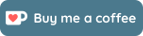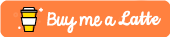# Showing Code in Latex ExamDesign class

ExamDesign is a Latex class that is used to create tests and exams. It supports a variety of question types such as True or False, Multiple Choice, and Fill-in the blanks. One of the best features of the class is the ability to scramble the order of the questions and the auto generated answer key. It makes creating multiple versions of a test a breeze.

If you are a Computer Science teacher, one thing you probably do on your test is to add some code for the students to analyze. A good Latex package to use would be the Listings package. Listings supports a whole variety of languages and is quite easy to use.

To use the Listings package with ExamDesign, you can not use a normal listings environment. Instead, you must put your section of code in a separate file and use the \lstinputlisting command. Ensure that your code files are in the same directory.

For example, if I wanted to add a short answer section where where the student figures out the output of a certain pieces of code, it would look something like this:

\begin{shortanswer}[title={\textbf{Program Tracing (1 mark each)}}, rearrange=yes]
\begin{question}
What is the output of the following program?	\hspace*{2.5in}Output: \rule{1in}{.4pt}
\lstinputlisting[frame=single]{code_trace01.txt}
\end{question}

\begin{question}
What is the output of the following program?	\hspace*{2.5in}Output: \rule{1in}{.4pt}
\lstinputlisting[frame=single]{code_trace02.txt}
\end{question}

\begin{question}
What is the output of the following program?	\hspace*{2.5in}Output: \rule{1in}{.4pt}
\lstinputlisting[frame=single]{code_trace03.txt}
\end{question}


You can also use the \lstinputlisting command in multiple choice questions as shown in the example below.

\begin{question}
The following program calculates the sum of numbers from 1 to n.
\lstinputlisting[frame=single,basicstyle=\ttfamily\footnotesize]{mc03.txt}
Which program below is equivalent?
\choice{\lstinputlisting[frame=single,basicstyle=\ttfamily\footnotesize]{mc03A.txt}}

\choice[!]{\lstinputlisting[frame=single,basicstyle=\ttfamily\footnotesize]{mc03B.txt}}

\choice{\lstinputlisting[frame=single,basicstyle=\ttfamily\footnotesize]{mc03C.txt}}

\choice{\lstinputlisting[frame=single,basicstyle=\ttfamily\footnotesize]{mc03D.txt}
}

\choice{\lstinputlisting[frame=single,basicstyle=\ttfamily\footnotesize]{mc03E.txt}
}
\end{question}


Hope that helps.

## Support

If you like my work, a small donation or feedback will be much appreciated.